# How to calculate for the air freight chargeable weight

How do I calculate the air freight chargeable weight of shipments? Is it the same with airfreight volumetric weight? What is its difference with dimensional weight?

We have to admit. We receive many calls about the “mysterious” chargeable weight and we understand why it could be a bit confusing to many. Thus the need for a simple guide that will help explain how to calculate the chargeable weight of air freight shipments.

## Why do shippers calculate on the basis of air freight chargeable weight?

Before we start exploring the calculation of air freight chargeable weight, let us first learn what it is and why is it necessary?

Air freight chargeable weight is different from volumetric or dimensional and gross weight. Rather it is the amount of weight charged on your invoice, which can either be the gross weight or the volumetric weight of your shipment

So what’s the difference between these two measurements?

### What is gross weight?

Gross (Actual) weight is also referred to as total weight of the cargo. This includes the weight of the shipment and the pallet.

#### How to calculate the gross weight of air freight shipments?

In the USA, most of the measurements are in imperial units such as pounds (lbs). Thus after getting the sum of the shipment weight and the pallet, you still need to convert this into kilograms as shown in the image below: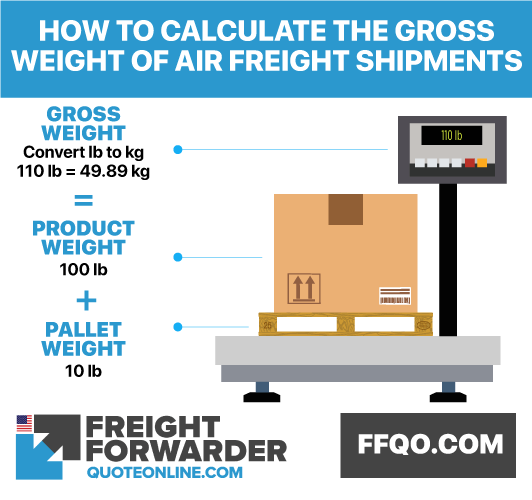How to calculate for the gross weight of shipments

### What is airfreight volumetric weight?

The airfreight volumetric weight or dimensional weight is an estimated weight value based from the length, width and height of a package. It is a theoretical weight calculated using a minimum air cubic conversion factor (167) chosen by the freight carrier. Note that when using the air cubic conversion factor of 167 that volume and weight need to be metric. That is cubic meters for volume and kilograms for weight.

#### How to calculate the airfreight volumetric weight formula?

To calculate the airfreight volumetric weight, follow the below formula: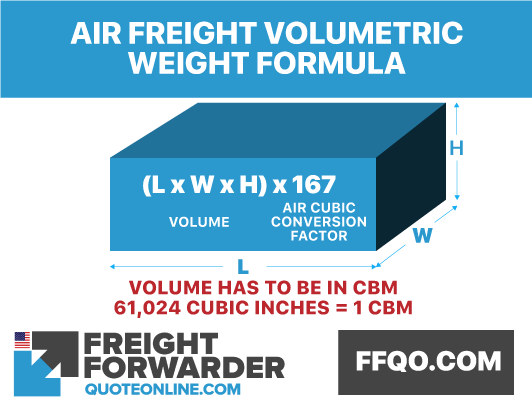How to calculate for the airfreight volumetric weight

### Understanding the difference between gross weight and volumetric weight

Volumetric weight is focused on measuring the space used by the shipment while gross weight is more focused on the lightness or heaviness of the package. It is best illustrated using the below illustration: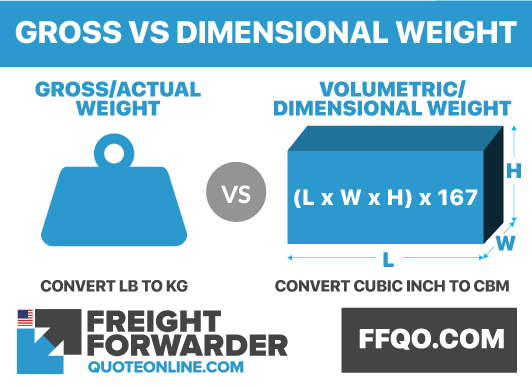Gross vs Dimensional Weight

### Why is there a need to calculate for the air freight chargeable weight of air freight shipments?

You must be thinking, why is there a need to have two different measurements in knowing the air freight chargeable weight of your shipment. It rests on the below principle.

Imagine a shipment of cotton and steel. It is quite obvious that a cotton shipment will occupy more space compared to a steel shipment. However, a steel shipment will be weighing more. Should both be charged using volume? Weight?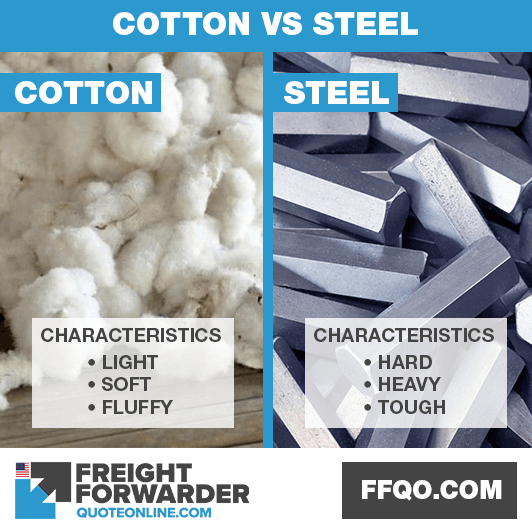Cotton vs steel characteristics

They choose the higher value between the volumetric weight and the actual weight of the cargo. This is fair enough because cotton shipments will occupy more space while steel shipments will weigh more. In both cases, shippers will be choosing the measurement with greater value.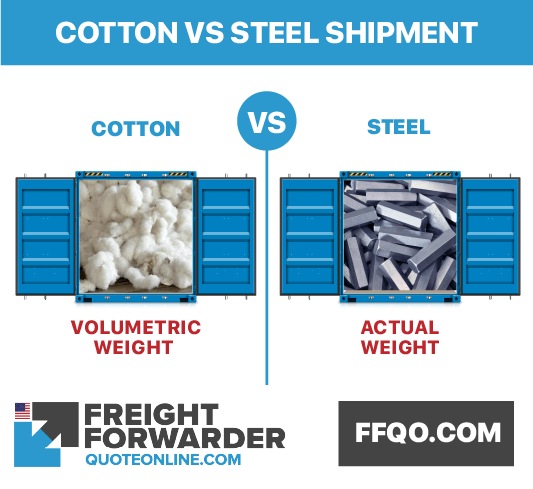Air freight chargeable weight difference of cotton and steel

## Steps on how to calculate the air freight chargeable weight

The following example explains how the air freight chargeable weight is to be calculated:

### Air Freight Shipment 1: Regularly shaped shipment ( Gross weight in kg> volumetric weight )

The gross weight of an air shipment can be higher in value compared to its volumetric weight. This makes it the chosen chargeable weight for the cargo as shown in the below example.

Shipment measurement:

• Length (l) = 40.16in
• Width (w) = 38.58in
• Height (h) = 41.73in
• Gross Weight (g) = 1653.47lbs

Step 1: Calculate the volume of the air freight shipment

Volume = length X width X height
= 40.16in x 38.58in x 41.73in
= 64655.33 cubic inches

Step 2: Convert the imperial measurement (cubic inches) into cubic meters
To convert cubic inches into cubic meters, follow this formula: 61,024 cubic inches = 1 cbm

Thus: 64655.33 cubic inches = 1.06cbm

Step 3: Finalise the volumetric weight
Air cubic conversion factor = 167
Volumetric weight = CBM X 167
= 1.06cbm x 167
= 177kgs

Step 4: Convert weight into kilograms
To convert pounds (lbs) into kilograms, follow this formula: 1 lbs = 0.4536kg

Gross weight is 750kg

Step 5: Compare the gross weight with the volumetric weight

Gross weight vs volumetric weight = 750 kgs vs 177kgs

Chargeable weight is the greater value which is 750 kgs.Air freight chargeable weight of calculation if gross weight is > volumetric weight

### Air Freight Shipment 2: Regularly shaped shipment 2pcs( Gross weight in kg < volumetric weight )

At times, the volumetric weight becomes of bigger value than the gross weight. In this instance, the volumetric weight is chosen as the chargeable weight.

Shipment measurement:

• Length (l) = 47.25 in
• Width (w) = 63 in
• Height (h) = 45.28 in
• Gross Weight (g) = 1102 lbs
• Number of boxes =2

Step 1: Calculate the volume of the air freight shipment

Volume  = (length X width X height) x 2
= (47.25in x 63in x 45.28in) x 2
= 269574.48 cubic inches

Step 2: Convert the imperial measurement into cubic meters
To convert cubic inches into cubic meters, follow this formula: 61,024 cubic inches = 1 cbm

Thus: 269574.48 cubic inches = 4.42cbm

Step 3: Finalise the volumetric weight
Air cubic conversion factor = 167
Volumetric weight = CBM X 167
= 4.42cbm x 167
= 738.14 kgs

Step 4: Convert weight into kilograms
To convert pounds (lbs) into kilograms, follow this formula: 1 lbs = 0.4536kg

Thus 1102 lbs = 500kgs

Step 5: Compare the gross weight with the volumetric weight

Gross weight vs volumetric weight = 500 kgs vs 738.14kgs

Chargeable weight is the greater value which is 738.14 kgs.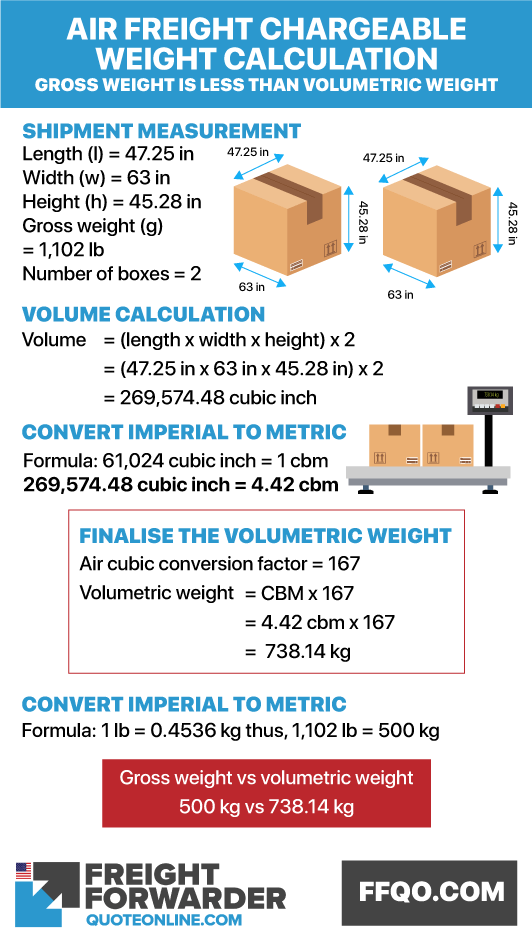Air freight chargeable weight calculation if volumetric weight is > gross weight

## How to calculate the air freight chargeable weight of an irregularly shaped container

There are instances wherein the item that has to be shipped is irregularly shaped. How is this to be calculated?

It is calculated in the same manner, however you need to know the right measurements of your shipment as illustrated in this image: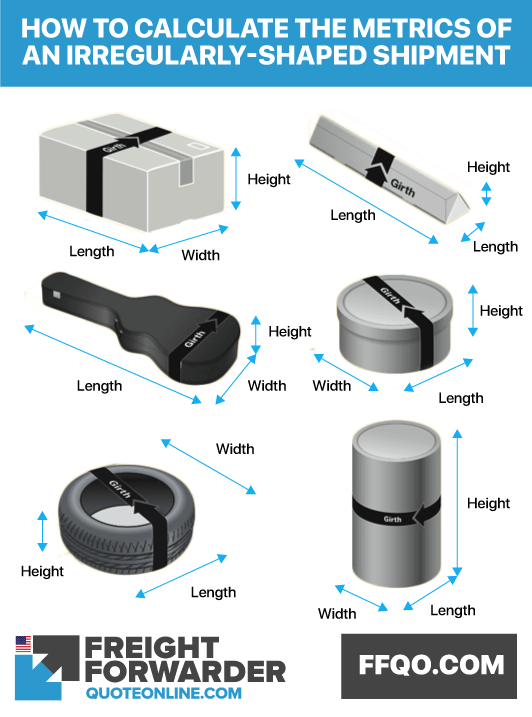How to calculate chargeable weight of irregularly shaped objects

After listing the measurements, you may now use the formula or air freight as discussed above.

Use our easy to use online quote tool otherwise, send us a message for assistance.

Do you need assistance moving your goods either via air? We are happy to help you. We run a fast, lean and transparent air freight service, so contact us online and send a message. You can even message us on Facebook for a quote on doing your air freight.